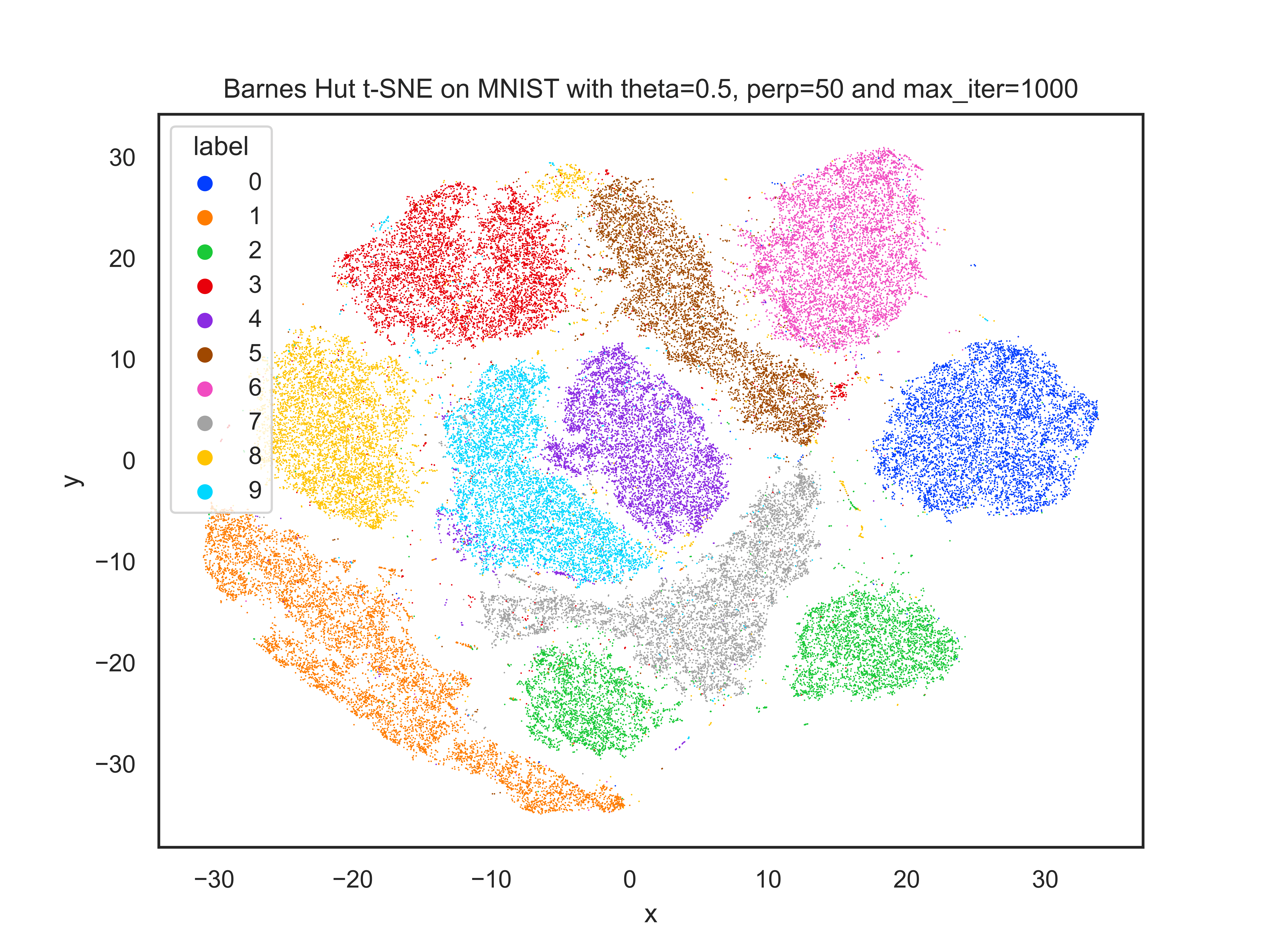## bhtsne

Exact and Barnes-Hut implementations of t-SNE

### 9 unstable releases

 0.5.1 Feb 13, 2022 Oct 15, 2021 Jul 24, 2021 Mar 21, 2021 Aug 21, 2020

#15 in Machine learning

Used in linfa-tsne

80KB
1.5K SLoC

# bhtsne

Parallel Barnes-Hut and exact implementations of the t-SNE algorithm written in Rust. The tree-accelerated version of the algorithm is described with fine detail in this paper by Laurens van der Maaten. The exact, original, version of the algorithm is described in this other paper by G. Hinton and Laurens van der Maaten. Additional implementations of the algorithm, including this one, are listed at this page.

## Installation

Add this line to your `Cargo.toml`:

``````[dependencies]
bhtsne = "0.5.1"
``````

### Documentation

The API documentation is available here.

### Example

The implementation supports custom data types and custom defined metrics. For instance, general vector data can be handled in the following way.

`````` use bhtsne;

const N: usize = 150;         // Number of vectors to embed.
const D: usize = 4;           // The dimensionality of the
// original space.
const THETA: f32 = 0.5;       // Parameter used by the Barnes-Hut algorithm.
// Small values improve accuracy but increase complexity.

const PERPLEXITY: f32 = 10.0; // Perplexity of the conditional distribution.
const EPOCHS: usize = 2000;   // Number of fitting iterations.
const NO_DIMS: u8 = 2;        // Dimensionality of the embedded space.

// Loads the data from a csv file skipping the first row,
// treating it as headers and skipping the 5th column,
// treating it as a class label.
// Do note that you can also switch to f64s for higher precision.
let data: Vec<f32> = bhtsne::load_csv("iris.csv", true, Some(&), |float| {
float.parse().unwrap()
})?;
let samples: Vec<&[f32]> = data.chunks(D).collect();
// Executes the Barnes-Hut approximation of the algorithm and writes the embedding to the
// specified csv file.
bhtsne::tSNE::new(&samples)
.embedding_dim(NO_DIMS)
.perplexity(PERPLEXITY)
.epochs(EPOCHS)
.barnes_hut(THETA, |sample_a, sample_b| {
sample_a
.iter()
.zip(sample_b.iter())
.map(|(a, b)| (a - b).powi(2))
.sum::<f32>()
.sqrt()
})
.write_csv("iris_embedding.csv")?;
``````

In the example euclidean distance is used, but any other distance metric on data types of choice, such as strings, can be defined and plugged in.

## Parallelism

Being built on rayon, the algorithm uses the same number of threads as the number of CPUs available. Do note that on systems with hyperthreading enabled this equals the number of logical cores and not the physical ones. See rayon's FAQs for additional informations.

## MNIST embedding

The following embedding has been obtained by preprocessing the MNIST train set using PCA to reduce its dimensionality to 50. It took approximately 3 minutes and 6 seconds on a 2.0GHz quad-core 10th-generation i5 MacBook Pro.~4MB
~69K SLoC